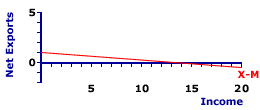Saturday  January 28, 2023
 AmosWEB means Economics with a Touch of Whimsy!YIELD TO MATURITY: The annual rate of return on a financial asset that is held until maturity. Yield to maturity depends on both the coupon rate and the face or par value paid at maturity. If the selling price of a financial asset is equal to its par value, then the yield to maturity is equal to the current yield and the coupon rate. However, if the asset is selling at a discount, then the yield to maturity exceeds the current yield, which is greater than the coupon rate. And if the asset is selling at a premium, then the yield to maturity is less than the current yield, which is below than the coupon rate.SLOPE, NET EXPORTS LINE:

The negative slope of the net exports line is based on the marginal propensity to import (MPM). Because net exports are exports minus imports, the induced change in imports causes an opposite change in net exports. As such, the slope of the net exports line is negative, less than zero (but greater than negative one). The slope of the net exports line affects the slope of the aggregate expenditures line and thus also affects the magnitude of the multiplier process.
Net Exports LineThe net exports line shows the relation between net exports by the foreign sector and the level of aggregate domestic income or production. The income and production measures most commonly used are national income and gross domestic product.

A representative net exports line is presented in the exhibit to the right. This red line, labeled X-M in the exhibit, is negatively sloped, indicating that greater levels of income or production generate smaller levels of net exports by the foreign sector. This negative relation indicates that imports, which are subtracted from exports to derived net exports, are induced by an expanding economy.

The net exports line graphically illustrates the net exports-income relation for the foreign sector, which is then added to the consumption line to derive the aggregate expenditures line used in Keynesian economics to identify equilibrium income and production.

The slope of the net exports line presented here is negative. In fact, the slope of the net exports line is numerically equal to the negative of the marginal propensity to import. In this case the slope is equal to -0.075. The negative slope reflects induced net exports--more income means fewer net exports. Click the [Slope] button to illustrate.

To illustrate the equality between slope and the negative of the marginal propensity to import, consider the equations for each. The slope of the net exports line is specified as the "rise" over the "run." The rise is the change in net exports measured on the vertical axis and the run is the change in income measured on the horizontal axis. However, because exports are autonomous, the only income induced change in net exports comes from imports, in a negative way.

 slope = riserun = change in net exportschange in income = - change in importschange in income
The marginal propensity to import (MPM) is the incremental change in net exports resulting from an incremental change in income.
 MPM = change in importschange in income
The negative slope of the net exports line is the marginal propensity to import, or the slope of the net exports line is the negative of the marginal propensity to import, on is the negative of the other.

The negative slope of the net exports line reflects induced net exports, which is net exports that depend on the level of income. If the aggregate economy has more income, then the foreign sector is induced to undertake fewer net exports. Of course, a drop in aggregate income induces the foreign sector to increase net exports.

 <= SLOPE, LONG-RUN AGGREGATE SUPPLY CURVE SLOPE, PRODUCTION POSSIBILITIES CURVE =>Recommended Citation:

SLOPE, NET EXPORTS LINE, AmosWEB Encyclonomic WEB*pedia, http://www.AmosWEB.com, AmosWEB LLC, 2000-2023. [Accessed: January 28, 2023].

Check Out These Related Terms...

Or For A Little Background...

And For Further Study...
Search Again?GRAY SKITTERY[What's This?] Today, you are likely to spend a great deal of time surfing the Internet seeking to buy either a country wreathe or galvanized steel storage shelves. Be on the lookout for jovial bank tellers.Your Complete ScopeA communal society, a prime component of Karl Marx's communist philosophy, was advocated by the Greek philosophy Plato."Even a mistake may turn out to be the one thing necessary to a worthwhile achievement."-- Henry FordQRQuantitative RestrictionA PEDestrian's Guide Xtra CreditTell us what you think about AmosWEB. Like what you see? Have suggestions for improvements? Let us know. Click the User Feedback link.| | | | | | | | | | |
| | | |

Thanks for visiting AmosWEB